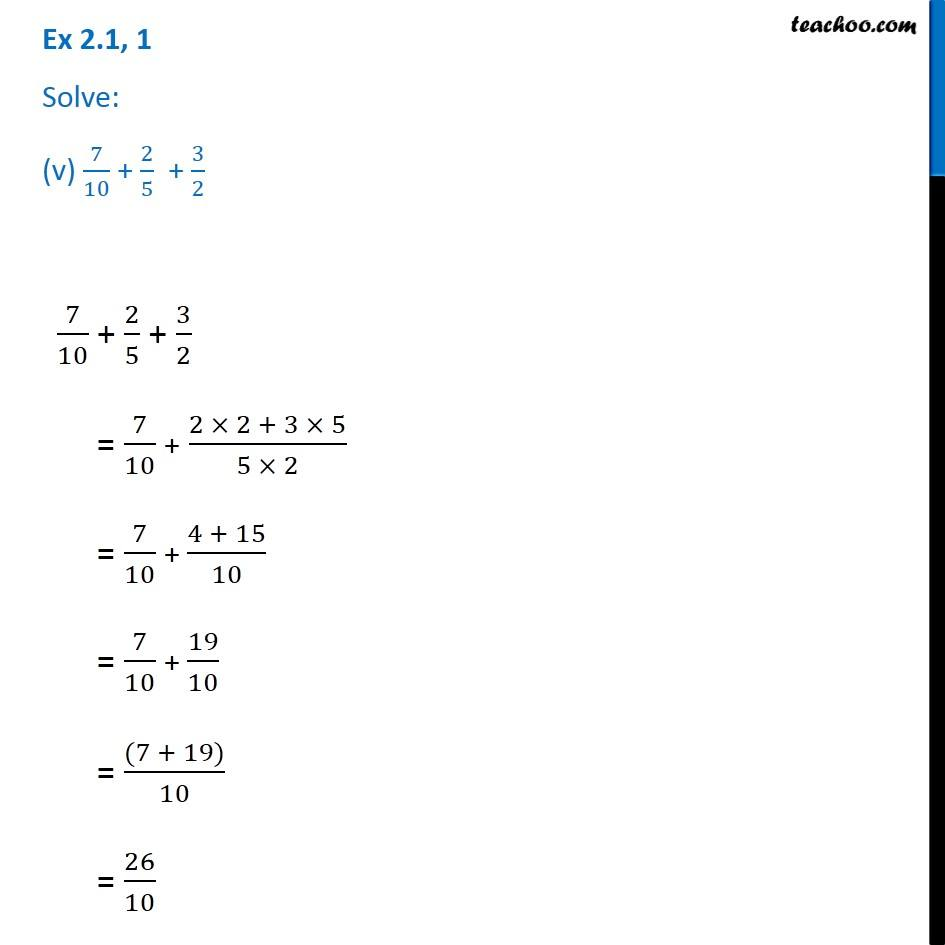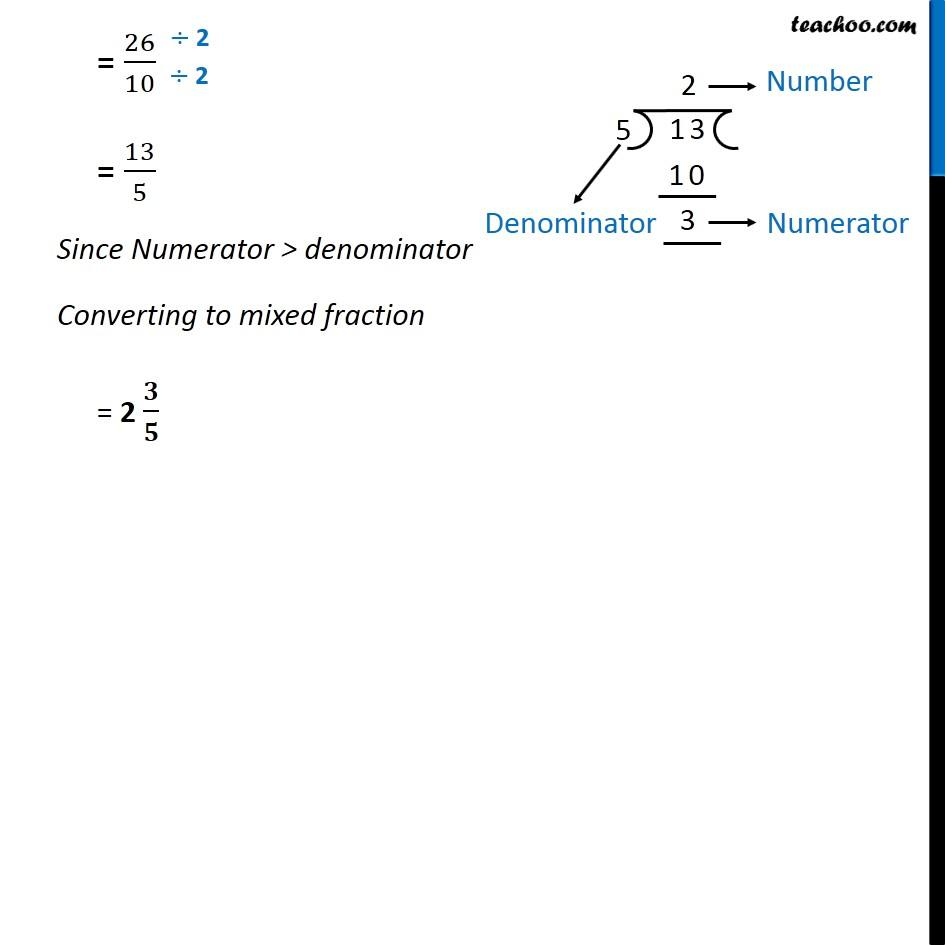Chapter 2 Class 7 Fractions and Decimals
Serial order wiseLearn in your speed, with individual attention - Teachoo Maths 1-on-1 Class

### Transcript

Question 1 Solve: (v) 7/10 + 2/5 + 3/27/10 + 2/5 + 3/2 = 7/10 + (2 × 2 + 3 × 5)/(5 × 2) = 7/10 + (4 + 15)/10 = 7/10 + 19/10 = ((7 + 19))/10 = 26/10 = 26/10 = 13/5 Since Numerator > denominator Converting to mixed fraction = 2 𝟑/𝟓